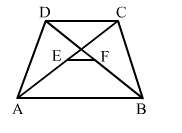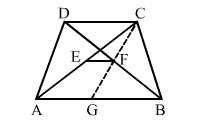# In a trapezium ABCD, if E and F be the mid-point of the diagonals AC and BD respectively

Question:

In a trapezium ABCD, if E and F be the mid-point of the diagonals AC and BD respectively. Then, EF = ?

(a) $\frac{1}{2} A B$

(b) $\frac{1}{2} C D$

(c) $\frac{1}{2}(A B+C D)$

(d) $\frac{1}{2}(A B-C D)$Solution:

(d) $\frac{1}{2}(A B-C D)$

Explanation:Join CF and produce it to cut AB at G.
Then ∆CDF  ≅ GBF                [∵ DF = BF, ​DCF = ​BGF and ​CDF = ​GBF]
∴ CD = GB
Thus, in
∆​CAG, the points E and F are the mid points of AC and CG, respectively.

$\therefore E F=\frac{1}{2}(A G)=\frac{1}{2}(A B-G B)=\frac{1}{2}(A B-C D)$